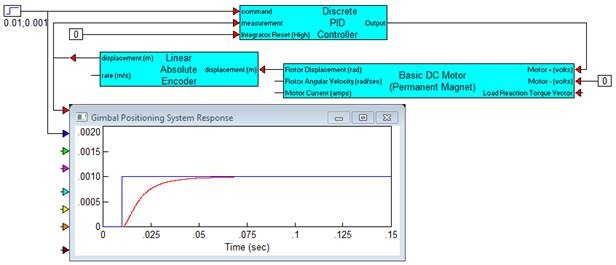##### AC Induction Motor DQ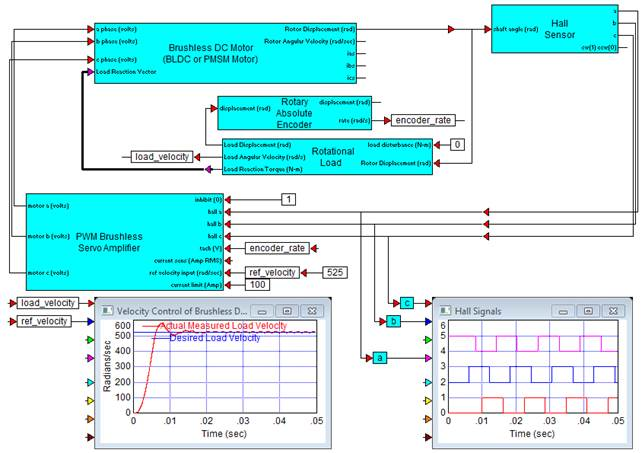Category: eMotors > Motors

The AC Induction Motor DQ block is derived from the system of nonlinear differential equations describing electromechanical motion of a 3-phase AC induction motor written in the arbitrary reference frame and reduced to the stationary frame by setting the frame angular velocity equal to 0. Input requires direct-quadrature (DQ) voltages and a load reaction torque vector if external mechanical load is used.

Mechanical dynamics include typical parameters, such as rotor shaft inertia and viscous friction. In addition, nonlinear dissipative factors, including Coulomb friction and stiction models are provided. The block can operate stand-alone to produce output displacement or velocity of the motor alone, or when a Rotation Load block is connected, combined dynamic response. When connected to the Rotation Load block, dynamic parameters are reflected back and combined with the motor dynamics by the linkage ratio. The linkage ratio is specified in the Rotation Load block. This creates proper dynamic motion of the combined motor-load connection. To connect the AC Induction Motor (DQ) block to the Rotation Load block, the motor displacement and load reaction vector connections from each block must be wired together.

Rotor shaft position, velocity and stator DQ phase currents are provided for sensor connections in monitoring and feedback applications.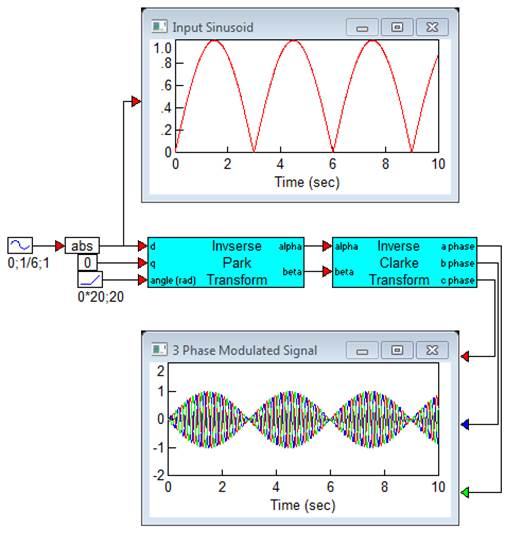Number of Motor Poles: Requires the number of motor pole pairs.

Stator Resistance (per phase): Requires the stator per phase coil resistance in ohms.

Stator Inductance (per phase): Requires the stator per phase coil inductance in henries.

Stator Leakage Inductance: Requires the specified stator leakage inductance in henries.

Rotor Resistance: Requires the rotor winding resistance in ohms.

Rotor Leakage Inductance: Requires the specified rotor leakage inductance in henries.

Rotor Moment of Inertia: Requires the moment of inertia of the rotor with respect to the axis of rotation in kg-m2.

Rotor Shaft Coulomb Friction Magnitude: Allows specification of constant directional dissipative force (Coulomb model) in units of N-m.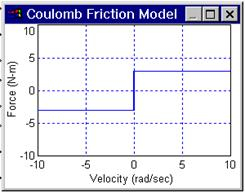Rotor Shaft Stiction Factor: Allows specification of a stiction force value or break-away torque. This parameter is normally not specified by the motor manufacturer, but can be obtained experimentally. Units are in N-m.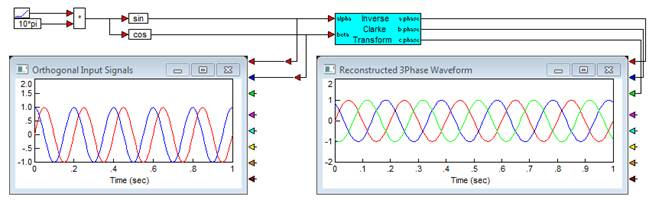Rotor Shaft Viscous Damping Factor: Requires the factor that linearly relates viscous damping force to angular velocity. This parameter is normally not specified by the motor manufacturer, but can be determined experimentally. Units are kg-m2/s.

#### Example

Diagram name: ACIM DQ Model <tbs. change name to AC Induction Motor DQ?>

Location: Examples > eMotors > AC Induction

A DQ frame voltage source is created using a 3-phase source, and Clarke and Park’s transforms (see example under Park Transform). Rotor displacement is connected to the Rotational Load block. Reaction torques are fed back to the motor model. DQ transient currents are observed by plotting signals from the output wires provided on the motor model.